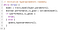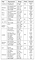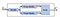# Oracle’s AutoML: What it is and how it works

Machine learning made possible with zero background

Machine learning has been the foreground of the rising popularity of data-driven software systems. However machine learning has a comparative high entry barrier. Shortage of Machine Learning expertises could potentially prevent many companies from benefiting from Machine Learning. Facing such challenges, both the academics and the industries have invested heavily in automating the machine learning process. Oracle’s AutoML is one such example.

# The classic machine learning process

The machine learning process usually starts with data collection and preprocessing. We then need to select an algorithm from a vast amount of possible machine learning algorithms. Some examples are logistic regression, random forest, support vector machine, convolution neural network and currently-popular transformer. After an algorithm is selected, we need to optimize the hyper-parameters and parameters of the algorithm. This process will be repeated until we find a model with good performance.

This approach has a few shortcomings. In this article, we will focus on two of them. First, it requires one to be familiar with many different machine learning algorithms and their use cases. The best machine learning model one can get heavily depends on his/her knowledge of machine learning. The whole process is pragmatic and experience-based. There are not many systematic approaches on how to select a model for a given dataset.

There are theoretic results on machine learning, such as how many data one needs to have in order for a model to be learnable. However those upper bounds are usually not tight enough to be useful.

The second problem is that hyper-parameter optimization is super time-consuming. Hyper-parameter optimization can be roughly described as the code in the figure below. The model training, line 3, can take hours or even days for large datasets and large models. It would be extremely expensive to even explore a tiny fraction of possible hyper-parameters. Therefore it takes experiences and luck to guess what hyper-parameters are good and to examine in the algorithm below.Hyper-parameter optimization

# Oracle AutoML

Oracle AutoML solves two problems aforementioned by automating the algorithm selection and accelerating hyper-parameter optimization.

The core idea behind the automated algorithm selection is a proxy model. A proxy model is an instance of the algorithm. The hyper-parameter is chosen such that the performance of the proxy model is representative of best possible performance of all the models of the algorithm on a dataset.

The figure below lists the proxy models for different algorithms. Let’s take AdaBoost Algorithm for an example. There are two hyper-parameters for this algorithm, learning_rate and n_estimators. Each combination of the hyper-parameter gives us a model of that algorithm. One model instance of Adaboost algorithm could have hyper-parameter, 1 for learning_rate and 2 for n_estimators while another model instance could have 2 and 2. The proxy model for Adaboost algorithm is one with hyper-parameter 0.0513 and 50.Proxy models for different algorithms

Once we have the proxy models, algorithm selection becomes trivial. We just run the proxy models on the training data and select the algorithm with best performance.Model selection with AutoML

Straggler is a serious issue in the hyper-parameter optimization. Traditionally hyper-parameter optimization is done in batch, as shown in figure a. We select P sets of hyper-parameters and evaluate those hyper-parameters in parallel. We then wait until all evaluations to complete and calculate next P sets of hyper-parameters to examine.

The problem with this approach is that if there is one evaluation runs slowly, the straggler, we need to wait for it even though all other P-1 evaluations have completed. There is a huge waste of resources. What AutoML did is that it makes the optimization asynchronous, figure b. Whenever one evaluation completes, we immediately compute the next hyper-parameter to examine and kicks off next round of evaluation. The pipeline is now fully utilized.

# Limitations of AutoML

There are also some limitations with the Oracle AutoML. It heavily relies on a proxy model to predict the performance of all the models of an algorithm. While it could work on models like logistic regression and support vector machine, it could be nearly impossible to have a proxy model for neural networks. The reason is that neural networks could approximate any functions/ algorithms. If we can have a proxy model for neural networks, we will have a proxy model for all models!

# Reference

1. Yakovlev, Anatoly, et al. “Oracle AutoML: a fast and predictive AutoML pipeline.” Proceedings of the VLDB Endowment 13.12 (2020): 3166–3180.

80% System; 20% ML